Forward Propagation In Neural NetworksIn this blog, we will intuitively understand how a neural network functions and the math behind it with the help of an example. In this example, we will be using a 3-layer network (with 2 input units, 2 hidden layer units, and 2 output units). The network and parameters (or weights) can be represented as follows.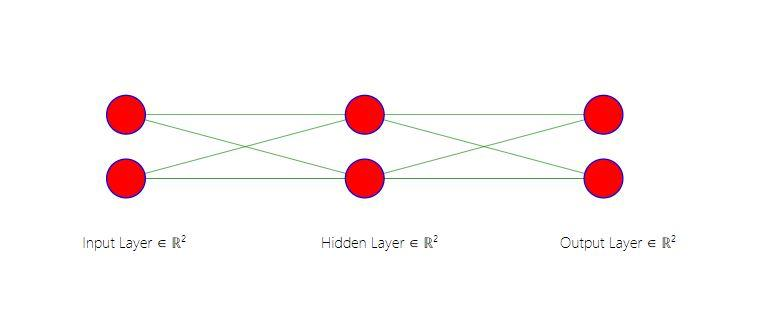Let us say that we want to train this neural network to predict whether the market will go up or down. For this, we assign two classes Class 0 and Class 1. Here, Class 0 indicates the datapoint where the market closes down, and conversely, Class 1 indicates that the market closes up. To make this prediction, a train data(X) consisting of two features x1, and x2. Here x1 represents the correlation between the close prices and the 10- day simple moving average (SMA) of close prices, and x2 refers to the difference between the close price and the 10-day SMA.

In this example below, the datapoint belongs to the class 1. The mathematical representation of the input data is as follows:

X = [x1, x2] = [0.85,.25] y= 

Example with two data points:The output of the model is categorical or a discrete number. We need to convert this output data also into a matrix form. This enables the model to predict the probability of a datapoint belonging to different classes. When we make this matrix conversion, the columns represent the classes to which that example belongs, and the rows represent each of the input examples.In the matrix y, the first column represents class 0 and second column represents class 1. Since our example belongs to the Class 1, we have 1 in the second column, and zero in the first.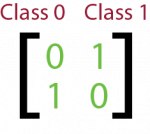This process of converting discrete/categorical classes to logical vectors/ matrix is called One Hot Encoding. We use one hot encoding as the neural network cannot operate on label data directly. They require all input variables and output variables to be numeric.

In a neural network learning, apart from the input variable we add a bias term to every layer other than the output layer. This bias term is a constant, mostly initialized to 1. The bias enables moving the activation threshold along the x-axis.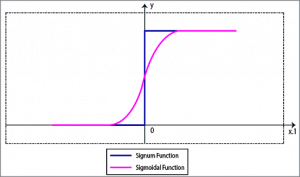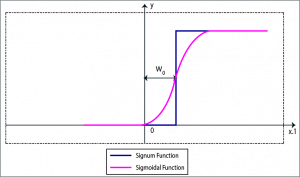When the bias is negative the movement is made to the right side, and when the bias is positive the movement is made to the left side. So a biased neuron should be capable of learning even such input vectors that an unbiased neuron is not able to learn. In the dataset X, to introduce this bias we add a new column denoted by ones, as shown below.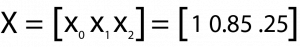Let us visualize the neural network, with the bias included in the input layer.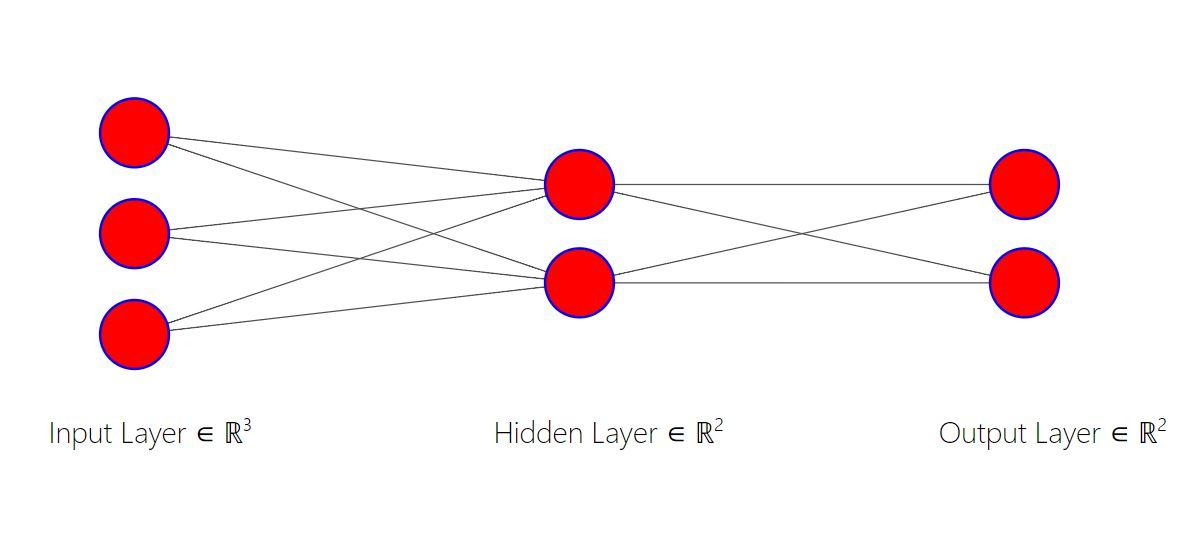The second layer in the neural network is the hidden layer, which takes the outputs of the first layer as its input. The third layer is the output layer which gives the output of the neural network.

Let us randomly initialize the weights or parameters for each of the neurons in the first layer. As you can see in the diagram we have a line connecting each of the cells in the first layer to the two neurons in the second layer. This gives us a total of 6 weights to be initialized, 3 for each neuron in the hidden layer. We represent these weights as shown below.Here, Theta1 is the weights matrix corresponding to the first layer.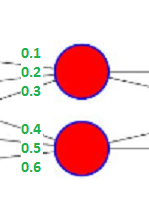The first row in the above representation shows the weights corresponding to the first neuron in the second layer, and the second row represents the weights corresponding to the second neuron in the second layer. Now, let’s do the first step of the forward propagation, by multiplying the input value for each example by their corresponding weights which is mathematically show below.

Theta1 * X

Before we go ahead and multiply, we must remember that when you do matrix multiplications, each element of the product, X*Theta, is the dot product sum of the row in the first matrix X with each of the columns of the second matrix Theta1.

When we multiply the two matrices, X and Theta1, we are expected to multiply the weights with the corresponding input example values. This means we need to transpose the matrix of example input data, X, so that the matrix will multiply each weight with the corresponding input correctly.z2 = Theta1*Xt

Here z2 is the output after matrix multiplication, and Xt is the transpose of X.

The matrix multiplication process:Let us say that we have applied a sigmoid activation after the input layer. Then we have to element-wise apply the sigmoid function to the elements in the z² matrix above. The sigmoid function is given by the following equation:

f(x) = 1/(1+e-x)

After the application of activation function, we are left with a 2x1 matrix as shown below.Here a(2) represents the output of the activation layer.

These outputs of the activation layer act as the inputs for the next or the final layer, which is the output layer. Let us initialize another random weights/parameters called Theta2 for the hidden layer. Each row in Theta2 represents the weights corresponding to the two neurons in the output layer.After initializing the weights (Theta2), we will repeat the same process that we followed for the input layer. We will add a bias term for the inputs of the previous layer. The a(2) matrix looks like this after the addition of bias vectors:Let us see how the neural network looks like after the addition of bias unit: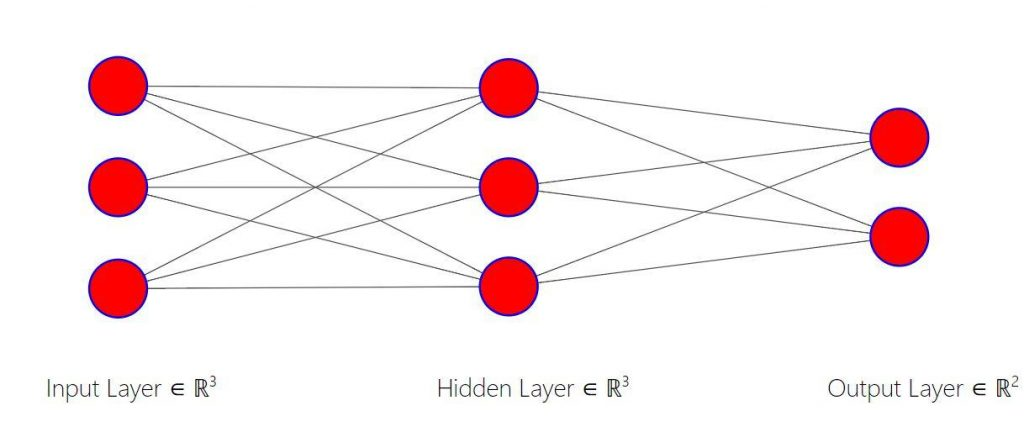Before we run our matrix multiplication to compute the final output z³, remember that before in z² calculation we had to transpose the input data a¹ to make it “line up” correctly for the matrix multiplication to result in the computations we wanted. Here, our matrices are already lined up the way we want, so there is no need to take the transpose of the a(2) matrix. To understand this clearly, ask yourself this question: “Which weights are being multiplied with what inputs?”.

Now, let us perform the matrix multiplication:

z3 = Theta2*a(2)

where z3 is the output matrix before the application of an activation function.

Here for the last layer, we will be multiplying a 2x3 with a 3x1 matrix, resulting in a 2x1 matrix of output hypotheses. The mathematical computation is shown below: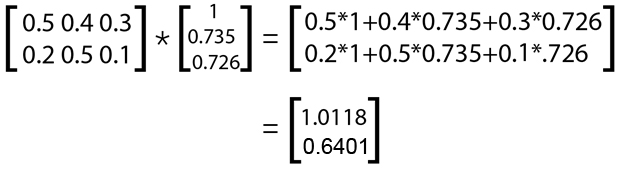After this multiplication, before getting the output in the final layer, we apply an element-wise conversion using the sigmoid function on the z² matrix.

a3 = sigmoid(z3)

Where a3 denotes the final output matrix.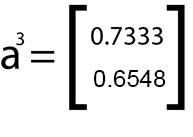The output of a sigmoid function is the probability of the given example belonging to a particular class. In the above representation, the first row represents the probability that the example belonging to Class 0 and the second row represents the probability of Class 1.

As you can see, the probability of example belonging to a Class 1 is lesser than Class 0, which is incorrect and needs to be improved.

In the next blog, we will discuss how to implement backward propagation to reduce the errors in the predictions. If you want to learn how to apply Neural Networks in trading then please check our new course Neural Networks & Deep Learning In Trading by Dr. Ernest P. Chan.

Disclaimer: All investments and trading in the stock market involve risk. Any decisions to place trades in the financial markets, including trading in stock or options or other financial instruments is a personal decision that should only be made after thorough research, including a personal risk and financial assessment and the engagement of professional assistance to the extent you believe necessary. The trading strategies or related information mentioned in this article is for informational purposes only.